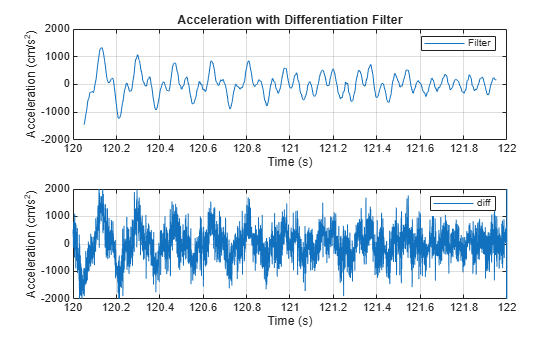Documentation

### This is machine translation

Mouseover text to see original. Click the button below to return to the English version of the page.

Note: This page has been translated by MathWorks. Click here to see
To view all translated materials including this page, select Country from the country navigator on the bottom of this page.

## Take Derivatives of a Signal

You want to differentiate a signal without increasing the noise power. MATLAB®'s function `diff` amplifies the noise, and the resulting inaccuracy worsens for higher derivatives. To fix this problem, use a differentiator filter instead.

Analyze the displacement of a building floor during an earthquake. Find the speed and acceleration as functions of time.

Load the file `earthquake`. The file contains the following variables:

• `drift`: Floor displacement, measured in centimeters

• `t`: Time, measured in seconds

• `Fs`: Sample rate, equal to 1 kHz

`load(fullfile(matlabroot,'examples','signal','earthquake.mat'))`

Use `pwelch` to display an estimate of the power spectrum of the signal. Note how most of the signal energy is contained in frequencies below 100 Hz.

`pwelch(drift,[],[],[],Fs)`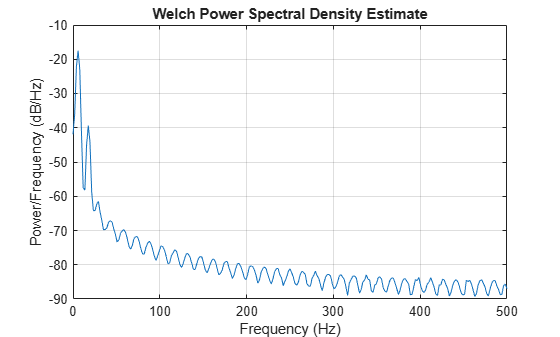Use `designfilt` to design an FIR differentiator of order 50. To include most of the signal energy, specify a passband frequency of 100 Hz and a stopband frequency of 120 Hz. Inspect the filter with `fvtool`.

```Nf = 50; Fpass = 100; Fstop = 120; d = designfilt('differentiatorfir','FilterOrder',Nf, ... 'PassbandFrequency',Fpass,'StopbandFrequency',Fstop, ... 'SampleRate',Fs); fvtool(d,'MagnitudeDisplay','zero-phase','Fs',Fs)```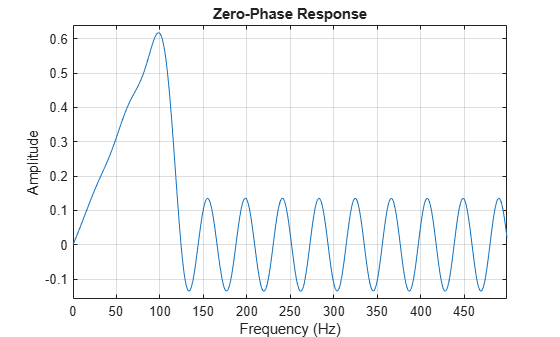Differentiate the drift to find the speed. Divide the derivative by `dt`, the time interval between consecutive samples, to set the correct units.

```dt = t(2)-t(1); vdrift = filter(d,drift)/dt;```

The filtered signal is delayed. Use `grpdelay` to determine that the delay is half the filter order. Compensate for it by discarding samples.

`delay = mean(grpdelay(d))`
```delay = 25 ```
```tt = t(1:end-delay); vd = vdrift; vd(1:delay) = [];```

The output also includes a transient whose length equals the filter order, or twice the group delay. `delay` samples were discarded above. Discard `delay` more to eliminate the transient.

```tt(1:delay) = []; vd(1:delay) = [];```

Plot the drift and the drift speed. Use `findpeaks` to verify that the maxima and minima of the drift correspond to the zero crossings of its derivative.

```[pkp,lcp] = findpeaks(drift); zcp = zeros(size(lcp)); [pkm,lcm] = findpeaks(-drift); zcm = zeros(size(lcm)); subplot(2,1,1) plot(t,drift,t([lcp lcm]),[pkp -pkm],'or') xlabel('Time (s)') ylabel('Displacement (cm)') grid subplot(2,1,2) plot(tt,vd,t([lcp lcm]),[zcp zcm],'or') xlabel('Time (s)') ylabel('Speed (cm/s)') grid```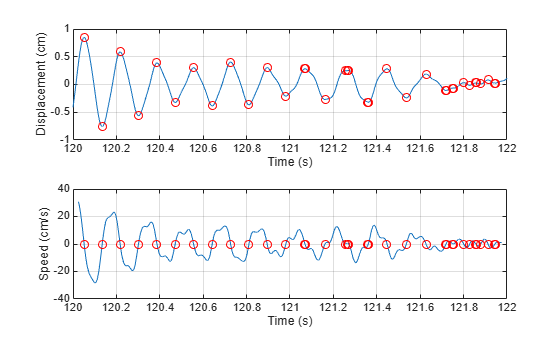Differentiate the drift speed to find the acceleration. The lag is twice as long. Discard twice as many samples to compensate for the delay, and the same number to eliminate the transient. Plot the speed and acceleration.

```adrift = filter(d,vdrift)/dt; at = t(1:end-2*delay); ad = adrift; ad(1:2*delay) = []; at(1:2*delay) = []; ad(1:2*delay) = []; subplot(2,1,1) plot(tt,vd) xlabel('Time (s)') ylabel('Speed (cm/s)') grid subplot(2,1,2) plot(at,ad) ax = gca; ax.YLim = 2000*[-1 1]; xlabel('Time (s)') ylabel('Acceleration (cm/s^2)') grid```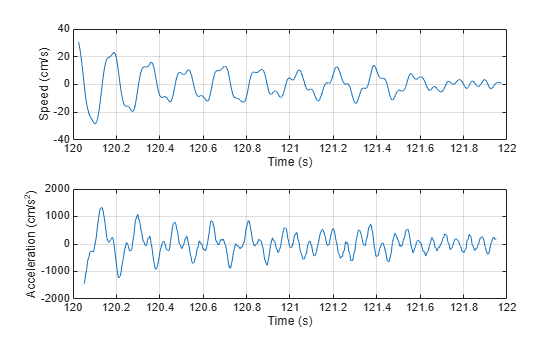Compute the acceleration using `diff`. Add zeros to compensate for the change in array size. Compare the result to that obtained with the filter. Notice the amount of high-frequency noise.

```vdiff = diff([drift;0])/dt; adiff = diff([vdiff;0])/dt; subplot(2,1,1) plot(at,ad) ax = gca; ax.YLim = 2000*[-1 1]; xlabel('Time (s)') ylabel('Acceleration (cm/s^2)') grid legend('Filter') title('Acceleration with Differentiation Filter') subplot(2,1,2) plot(t,adiff) ax = gca; ax.YLim = 2000*[-1 1]; xlabel('Time (s)') ylabel('Acceleration (cm/s^2)') grid legend('diff')```